# Time Delay and Scaling in Multirate DSP Filters

This example demonstrates the effect of time delay and scaling in multirate filters, and how to calculate them.

### Time Delay and Scaling Model in Multirate Filters

A multirate filter is a cascade combining upsampling, downsampling, and convolution filters (FIR or IIR). Such filter structures are often used to implement resampling systems, wherein the output is a resampled version of the input at a different rate. Informally, for an input $\mathit{u}\left(\mathit{n}\right)$, the output of a resampling system is $\mathit{y}\left(\mathit{k}\right)=\mathit{u}\left(\mathit{S}\cdot \mathit{k}-\mathit{D}\right)$. This is stated with a slight abuse of notations, since $\mathit{u}\left(\mathit{S}\cdot \mathit{k}-\mathit{D}\right)$ is undefined when $\mathit{S}\cdot \mathit{k}-\mathit{D}$ is noninteger. More formally, the input is interpolated to a continuous domain function$\mathit{f}\left(\mathit{t}\right)$ from which the output is sampled:

$\mathit{y}\left(\mathit{k}\right)=\mathit{f}\left(\frac{\mathit{k}}{{\mathrm{Fs}}_{\mathrm{out}}}-\mathit{D}\right)$.

The input can sometimes be approximated by sampling$\mathit{f}\left(\mathit{t}\right)$ at the inut rate $\mathit{u}\left(\mathit{n}\right)\approx \mathit{f}\left(\frac{\mathit{n}}{{\mathrm{Fs}}_{\mathrm{in}}}\right)$. Approximation is used instead of equality due to the filtering operation (e.g. lowpass/highpass) which is an integral part of the interpolation model. The interpolation model $\mathit{u}\left(\mathit{n}\right)↦\mathit{f}\left(\mathit{t}\right)$ does not necessarily preserve the values of every input $\mathit{u}\left(\mathit{n}\right)$.

The constants ${\mathrm{Fs}}_{\mathrm{in}}$, ${\mathrm{Fs}}_{\mathrm{out}}$ and $\mathit{D}$ in the equations above are the input sample rate, output sample rate, and the resampling output delay respectively. For many multirate filtering applications, it is useful to find ${\mathrm{Fs}}_{\mathrm{out}}$ and $\mathit{D}$ for a given input sample rate ${\mathrm{Fs}}_{\mathrm{in}}$.

### Using The `outputDelay` Function

You can use the `outputDelay` function to calculate the resampling output delay D and output sample rate `FsOut` for a given filter object operating at rate `FsIn`. This function is available for any DSP System object that supports filter analysis methods. For a list of supported objects, refer to the `outputDelay`. The returned delay value D is specified in the natural units of the interpolated signal (usually seconds), corresponding to the input sample rate.

Consider a signal $\mathit{u}\left(\mathit{n}\right)$ that is sampled from a continuous signal $\mathit{f}\left(\mathit{t}\right)$ at a rate of `Ts`=0.1`s` (that is, `Fs`=10 `Hz`). Add zero-mean white Gaussian noise with a standard deviation of 0.05.

```f = analyticSignal(); % x is a function of a real variable Ts = 1/10; n = (0:511)'; % Sample index vector u = f(n*Ts)+ 0.05*randn(size(n)); figure(Position = [0 0 900 200]) plot(n*Ts, u); xlabel('time'); legend('Input');```To eliminate the high frequency noise, design a lowpass filter and apply it to the signal. This lowpass has a cutoff at 15% of the Nyquist frequency with a transition width of 10%. Plot the input against the filtered output on the same graph. Note the delay between the input and the filtered signal.

```Fs = 1/Ts; Fnyq = Fs/2; F0 = 0.15; % Cutoff frequency normalized to the Nyquist frequency TW = 0.1; g1 = dsp.LowpassFilter(SampleRate = Fs, ... PassbandFrequency = (F0-TW/2)*Fnyq, ... StopbandFrequency = (F0+TW/2)*Fnyq); y = g1(u); ts = timescope(SampleRate=Fs, ... ChannelNames={'Input', 'Lowpass Output'},... YLimits=[-3,3]); ts(u, y)```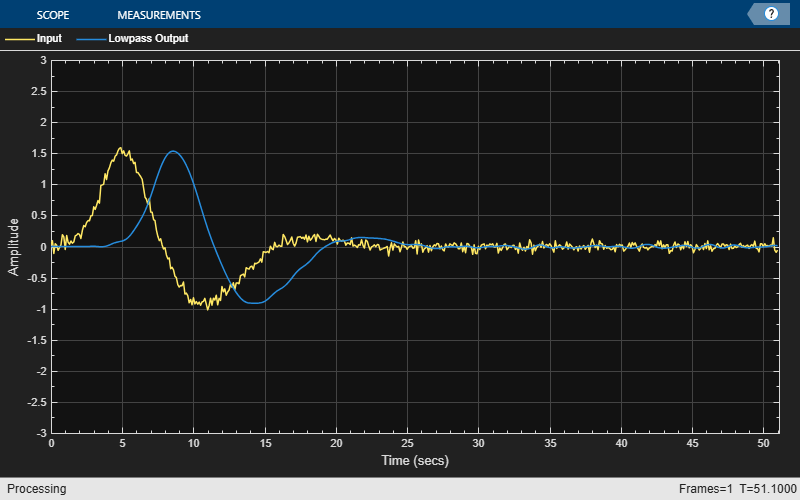The observed delay is inherent to any convolution filter, such as the one implemented in `dsp.LowpassFilter`. Call the `outputDelay` function to find that output delay.

`D = g1.outputDelay()`
```D = 3.6000 ```

To align the input with the output, shift the output back in time by D units, of shift the input forward in time by the same amount. You can perform such a shift using the `TimeDisplayOffset` property of the `timescope` object. When you specify a vector in `TimeDisplayOffset` instead of a scalar, each input channel of the `timescope` object has its own delay, corresponding to the entries of the vector in `TimeDisplayOffset`. Set the first channel (input) delay to D, and keep the second channel (output) with no delay. The two channels are now synchronized.

```release(ts); ts.TimeDisplayOffset = [D, 0]; ts(u, y)```The same delay can be used with standard MATLAB® plots.

```plot(n*Ts, u); hold on; plot(n*Ts-D, y); hold off; xlabel('time'); legend('Input', 'Output (filtered)');```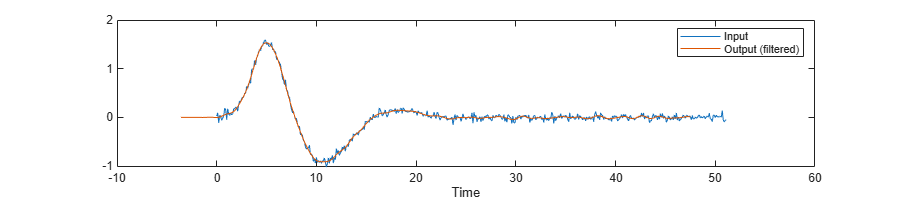#### The Relation Between Resampling Output Delay and the Group Delay

The `outputDelay` function uses the group delay of the convolution stages to calculate the overall resampling output delay. Although the two terms are closely related, there are several distinctions between the two:

• Scalar vs a function: Group delay is a function of frequency, defined as $\mathit{G}\left(\omega \right)=-{\varphi }^{\prime }\left(\omega \right)$ where $\varphi \left(\omega \right)$ is the phase response of a convolution system. Output delay is defined as a scalar, and it stems from the resampling model $\mathit{y}\left(\mathit{k}\right)=\mathit{f}\left(\mathit{k}/{\mathrm{Fs}}_{\mathrm{out}}-\mathit{D}\right)$.

• Scope of definition: Output delay applies to multirate filters, whereas group delay is well defined only for convolution systems (single-rate LTI systems).

• Units: Group delay is measured in sample units. Output delay is defined in time units of the interpolated signal $\mathit{f}\left(\mathit{t}\right).$

When the convolution stages of a multirate filter have a linear phase, their group delays do not depend on the input frequency. Symmetric filter designs, which are very common in DSP applications, have a linear phase. In the simple case of a single-stage symmetric convolution filter, the output delay is merely the (constant) group delay $\mathit{G}$ scaled to the sample time, i.e. $\mathit{D}=\frac{\mathit{G}}{\mathrm{Fs}}$. For a single-stage filter with a nonlinear phase, the output delay depends on the input frequency, namely $\mathit{D}\left(\mathit{f}\right)=\frac{1}{\mathrm{Fs}}\mathit{G}\left(\frac{\pi \mathit{f}}{\mathrm{Fs}}\right)$. For a multistage filter, the output delay is the sum of output delays of its stages. The multi-stage and nonlinear phase cases will be discussed later in this example.

In the simple case of a symmetric single-stage filter, the group delay can be thought of as the center of mass of its impulse response, which is its point of symmetry. The filter `g1` in the example above is a 73-taps FIR, symmetric about its 36th index.

```h = g1.impz(); stem(0:length(h)-1, h); hold on; xline(36, Color='red'); hold off; xlabel('n (samples)')```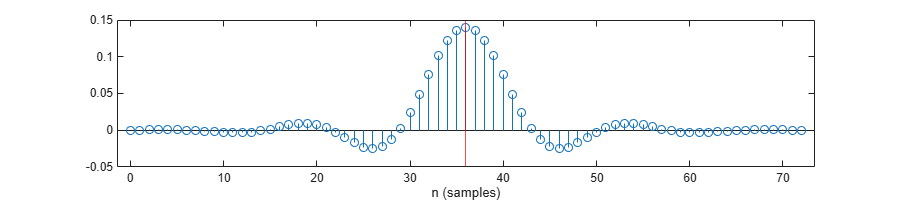The filter introduces a group delay of 36 samples, which is equivalent to an output delay of 3.6 time units accounting for the sample rate `Fs=`10 Hz. Calculate the output delay using center-of-mass weighted sum formula $\frac{{\sum }_{\mathit{k}=0}^{\mathit{L}-1}\mathit{k}\cdot \mathit{h}\left[\mathit{k}\right]}{{\sum }_{\mathit{j}=0}^{\mathit{L}-1}\mathit{h}\left[\mathit{j}\right]}$ and verify that it is indeed 3.6, the exact same value returned from `outputDelay`.

`D_cm = Ts*sum((0:length(h)-1)'.*h)/sum(h)`
```D_cm = 3.6000 ```

#### Working With Sample Rates

You can specify or override the input sample rate by using the named argument `FsIn`. For example, calculate the output delay assuming that the input sample rate is 2 kHz instead of 10 Hz. Note that the returned delay value changes accordingly to reflect the new time units.

`D2k = g1.outputDelay(FsIn = 2e3) % Override the sample rate to 2 kHz`
```D2k = 0.0180 ```

By default, `FsIn` is obtained from the object on which `outputDelay` is called. If the object has an intrinsic sample rate property, `FsIn` equals that property. For example, `dsp.LowpassFilter` and `dsp.IIRHalfbandDecimator` have the property `SampleRate`, and `dsp.FarrowRateConverter` has the property `InputSampleRate`. For objects that do not have a sample rate property, such as `dsp.FIRFilter` and `dsp.FIRDecimator`, the default value for `FsIn` is 1 (one sample per second).

#### Obtaining The Output Sample Rate of a Multirate Filter

Multirate filters such as `dsp.FIRRateConverter` often involve rate change, so the input sample rate and output sample rate are not equal. In the following example, the output appears shrank on the time domain.

```g2 = dsp.FIRRateConverter(7,16); y = g2(u); D = g2.outputDelay(FsIn = Fs); ts = timescope(SampleRate = Fs, ... TimeDisplayOffset = [0, -D],... ChannelNames={'Input', 'Output'},... YLimits=[-3,3]); ts(u, y);```The `outputDelay` function returns the output sample rate as the second ouput argument, which is particularly convenient when using `dsp.FilterCascade` objects. Call `outputDelay` with two output arguments to obtain ${\mathrm{Fs}}_{\mathrm{out}}$.

`[D, FsOut] = g2.outputDelay(FsIn = Fs)`
```D = 1.2000 ```
```FsOut = 4.3750 ```

You can specify different sample rates for each channel of a `timescope` object, by setting a vector to its `SampleRate` property instead of a scalar. Make sure to use the same channel order as you step through the `timescope` object. Set the first entry of `SampleRate` to `Fs` (the input sample rate), and the second to `FsOut` (the output sample rate). The input and output now have the same scale on the plot.

```ts = timescope(SampleRate = [Fs, FsOut], ... TimeDisplayOffset = [0, -D],... ChannelNames={'Input', 'Output'},... YLimits=[-3,3]); ts(u, y);```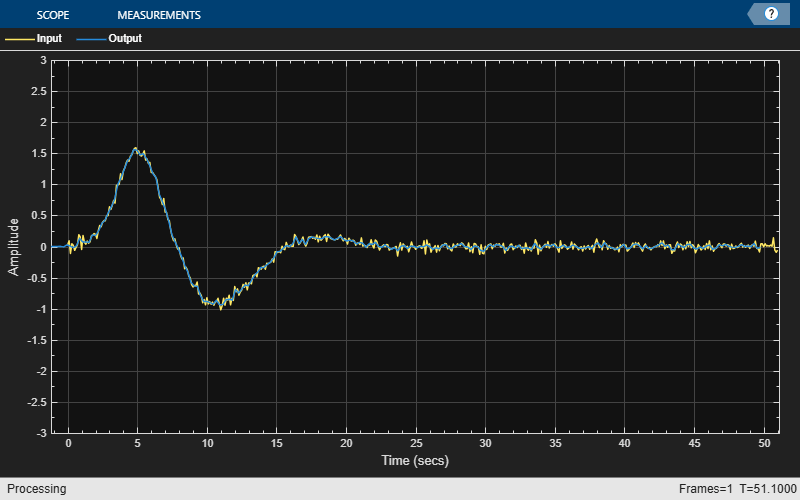### Bandlimited Mode: Using `outputDelay` with Nonlinear Phase Filters

So far, we used symmetric filter designs, which have a linear phase, and a constant group delay. Asymmetric filters, on the other hand, have a varying group delay and can exhibit signal distortion in the time domain, which breaks the resampling model described above. Using `outputDelay` in such cases is possible, but requires some caution. This is discussed in the next section.

#### Distortion in Nonlinear Phase Filters

Causal and stable rational IIR filters are common in DSP, and they often have a nonlinear phase. For example, consider the following lowpass IIR design, which clearly has a varying group delay.

```F0 = 0.35; TW = 0.2; g3 = dsp.LowpassFilter(SampleRate = Fs, ... PassbandFrequency = (F0-TW/2)*Fnyq, ... StopbandFrequency = (F0+TW/2)*Fnyq, ... FilterType='IIR'); [G, W] = g3.grpdelay(2048, Fs); plot(W, G); xlabel('Frequency (Hz)'); ylabel('Group Delay (samples)');```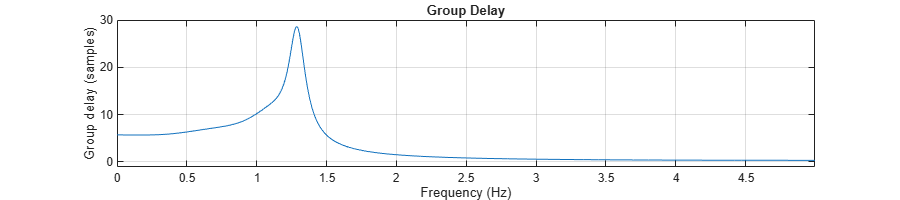The `outputDelay` function alerts the user if the system has a nonlinear phase convolution stage.

```>> D = g3.outputDelay(); % Throws a warning. % The System object has a nonlinear phase, but a carrier frequency Fc has not been specified. % To suppress this warning, specify Fc argument explicitly, or call the outputDelay method with % band measurement output. If you don't specify any value, the default carrier frequency is Fc=0. ```

To demonstrate the effect of a nonlinear phase distortion, input a short time pulse to the IIR filter `g3`. Note that the input and the output are not related by a time delay and scaling, i.e. they no longer satisfy the resampling model$\mathit{y}\left(\mathit{k}\right)=\mathit{f}\left(\mathit{k}/{\mathrm{Fs}}_{\mathrm{out}}-\mathit{D}\right)$ and $\mathit{u}\left(\mathit{n}\right)\approx \mathit{f}\left(\mathit{n}/{\mathrm{Fs}}_{\mathrm{in}}\right)$.

```v = f(10*Ts*n); y = g3(v); release(ts); ts.TimeDisplayOffset = [D, 0]; ts(v, y); ts.TimeSpanSource='Property'; ts.TimeSpan=20;```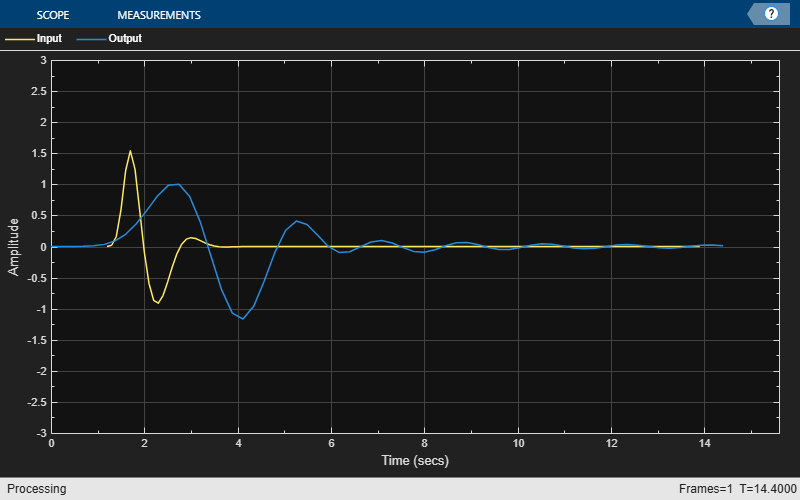Using `outputDelay` on a filter with nonlinear phase stages only makes sense if the group delay of the stages is relatively constant on the input signal band. That could happen if:

• The filter stages have a flat group delay response over a known band, or

• If the input is narrowband, so that the group delay response can be approximated as a constant on the input band.

In any case, since the group delay is not constant, you need to specify the frequency from which the group delay is sampled. The `outputDelay` function accepts this frequency (specified in input sample rate units) through the parameter `Fc`.

#### Nonlinear Phase Filters with a Partially Flat Group Delay Response (Quasi-Linear Phase)

Some filter designs have a nonlinear phase, yet still have a relatively flat group delay on subbands. For example, any FIR designed using the `designFracDelayFIR` function has a relatively flat group delay. Other examples include the `dsp.IIRHalfbandDecimator` and the `dsp.FIRHalfbandInterpolator` filters that are operating in the Quasi-linear phase design mode. The Quasi-linear design mode compensates for the nonlinear phase of the IIR on the passband and considerably reduces the distortion.

Design an IIR halfband decimator, and plot its group delay. The group delay is obviously not constant, yet relatively flat outside the transition band.

```g4 = dsp.IIRHalfbandDecimator(DesignMethod='Quasi-linear phase'); [G, W] = g4.grpdelay(8192, g4.SampleRate); plot(W, G); xlabel('Frequency (Hz)'); ylabel('Group Delay (samples)');```To obtain the output delay for a bandlimited signal on the flat region, set `Fc` to be any frequency within that flat region. Note that a slight deviation of the delay values of different frequencies within the flat region is normal and is expected.

`D1 = g4.outputDelay(Fc = 0)`
```D1 = 4.9884e-04 ```
```Fc = 5000; D2 = g4.outputDelay(Fc = Fc) % 5 kHz is still in the flat region```
```D2 = 4.9889e-04 ```

#### Input Band Measurement

For a filter with nonlinear phase stages, changing `Fc` alters the output delay D. The `outputDelay` function can calculate the interval of input frequencies `B = [f1, f2]` around `Fc` that have a delay value close to D up to a tolerance, or $|\mathit{D}\left(\mathit{f}\right)-\mathit{D}\left(\mathrm{Fc}\right)|<\frac{\mathrm{Tol}}{\mathrm{Fsin}}$ for every $\mathit{f}\in \left[\mathrm{f1},\mathrm{f2}\right]$. The edges of the band, `f1` and `f2, `are returned in the third output argument of the `outputDelay` function. The tolerance is specified in sample time units using the `Tol` named parameter. For example, obtain the input frequency band for which the delay deviates by up to `Tol` = 1 second from the nominal value D at the frequency `Fc`.

`[D, ~, B] = g4.outputDelay(Fc = Fc, Tol = 1) % Discard the FsOut output argument`
```D = 4.9889e-04 ```
```B = 1×2 103 × -9.9268 9.9268 ```

Note that the measured band is two-sided and might contain negative frequencies.

```plot(W, G); xlabel('Frequency (Hz)'); ylabel('Group Delay (samples)'); hold on; I = W>=B(1) & W<=B(2); % Find band indices plot(W(I), G(I), Color='m', LineWidth=3); plot(Fc, D*g4.SampleRate, 'ro'); legend('Delay', 'Input band', 'Fc') grid on hold off```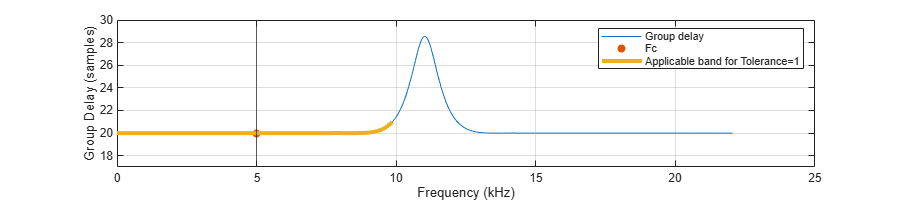#### Band Measurement Resolution

If the tolerance value of the band measurement is very low, the resulting band may be a singleton (contains a single frequency, the specified frequency `Fc`), which is a degenerate case. This is because the group delay search resolution is too coarse for the specified tolerance. For example, repeat the band measurement of the system `g4` with a tight tolerance of `Tol `= 1e-3. The returned B equals to `[Fc, Fc]`, indicating that is has a single frequency.

```Fc = 9600; Tol = 1e-3; [D, ~, B] = g4.outputDelay(Fc = Fc, Tol = Tol) ```
```D = 5.0657e-04 ```
```B = 1×2 9600 9600 ```
```plot(W, G,'-o'); xlabel('Frequency (Hz)'); ylabel('Group Delay (samples)'); hold on; plot(Fc, D*g4.SampleRate, 'ro'); yline(D*g4.SampleRate+[-Tol Tol], Color=[0.5 0 0.8]) legend('Delay', 'Fc', 'Tolerance interval') grid on hold off xlim([Fc-5 Fc+5]);```An obvious solution is to relax the tolerance. However, if you want to keep the low tolerance, you can instead increase the group delay resolution by using the `FFTLength` parameter. The default length is ${2}^{13}=8192$. Increase `FFTLength` to ${2}^{16}=65536$ and notice that the returned `B` has two distinct edges, and not a single frequency.

`[D, ~, B] = g4.outputDelay(Fc = Fc, Tol = Tol, FFTLength=2^16)`
```D = 5.0657e-04 ```
```B = 1×2 103 × 9.5998 9.6004 ```

To plot the band, calculate the group delay with increased resolution as well.

```[G, W] = g4.grpdelay(2^16, 'whole', g4.SampleRate); plot(W, G,'-o'); xlabel('Frequency (Hz)'); ylabel('Group Delay (samples)'); hold on; I = W>=B(1) & W<=B(2); % Find band indices plot(W(I), G(I), Color='m',LineWidth=3); plot(Fc, D*g4.SampleRate, 'ro'); yline(D*g4.SampleRate+[-Tol Tol], Color=[0.5 0 0.8]) legend('Delay','Input band','Fc','Tolerance interval'); grid on hold off xlim([Fc-5 Fc+5])```#### Narrowband Signals

The `outputDelay` function can be used even if the group delay is not flat, given that the input signal is a narrowband signal. The band measurement returned from `outputDelay` can be used to determine the maximal bandwidth for the signal subject to a delay tolerance.

When the system under consideration has a nonlinear phase and the signal is narrowband signal centered around some carrier frequency ${\omega }_{\mathit{c}}=2\pi \cdot \mathrm{Fc}$, the resampling model is slightly different than in the linear phase case. The input is approximated by

$\mathit{u}\left(\mathit{n}\right)\approx \mathit{f}\left(\mathit{n}\cdot {\mathrm{Ts}}_{\mathrm{in}}\right)\cdot \mathrm{cos}\left({\omega }_{\mathit{c}}\cdot \mathit{n}\cdot {\mathrm{Ts}}_{\mathrm{in}}\right)$,

and the output is

$\mathit{y}\left(\mathit{k}\right)\approx \mathit{f}\left(\mathit{k}\cdot {\mathrm{Ts}}_{\mathrm{out}}-\mathit{D}\right)\cdot \mathrm{cos}\left({\omega }_{\mathit{c}}\cdot \left(\mathit{k}\cdot {\mathrm{Ts}}_{\mathrm{out}}-{\mathit{D}}_{\mathit{p}}\right)\right)$.

The basedband signal is delayed by the output delay $\mathit{D}$, which is calculated using the group delay obtained from ${\omega }_{\mathit{c}}$. The carrier signal, however, experiences a different delay, called phase delay, and denoted ${\mathit{D}}_{\mathit{p}}$. For single-rate systems, the phase delay is merely the negative of the system's phase response measured at ${\omega }_{\mathit{c}}$ and divided by ${\omega }_{\mathit{c}}$.

${\mathit{D}}_{\mathit{p}}=-\frac{\angle \mathit{H}\left({\omega }_{\mathit{c}}\right)}{{\omega }_{\mathit{c}}}$ .

Consider the IIR design `g3`, and obtain the output delay for a narroband signal with the center frequency `Fc=0.75 Hz` . Indeed, the returned band `B=[0.526 Hz, 0.885 Hz]` contains the carrier frequency, and has a bandwidth of `0.3589 Hz`.

```Fc = 0.15*Fnyq; % Carrier frequency Tol = 1; [D, ~, B] = g3.outputDelay(FsIn = Fs, Fc=Fc, Tol = Tol) % Find deviation up to 1 time unit```
```D = 0.7457 ```
```B = 1×2 0.5261 0.8850 ```

Plot the response and the band, and verify that the frequency `Fc` is indeed contained in the band.

```[G, W] = g3.grpdelay(2048, Fs); plot(W, G); xlabel('Frequency (Hz)'); ylabel('Group Delay (samples)'); hold on; I = W>=B(1) & W<=B(2); % Find band indices plot(W(I), G(I), Color='m', LineWidth=3); Fc = 0.15*Fnyq; % Carrier frequency plot(Fc, D*Fs, 'ro'); yline(D*g3.SampleRate+[-Tol Tol], Color=[0.5 0 0.8]) legend('Delay','Input band','Fc','Tolerance interval'); grid on hold off```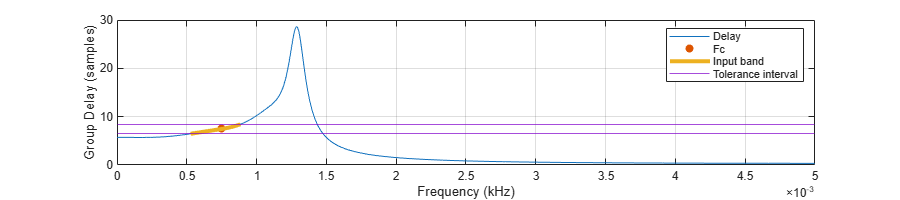#### Carrier Shift and Phase Delay

Filter a modulated signal through the filter, and plot the input against the output with the apropriate delay. As expected, the delayed input appears synchronized with the output under the same envelope (the delayed baseband signal), but have a slight phase shift - the phase delay of the carrier signal.

```ubase = f(n*Ts); % Baseband signal (evelope) wc = 2*pi*Fc; % Carrier frequency u = f(n*Ts).*cos(wc*n*Ts) + 0.05*randn(size(n)); y = g3(u); ts = timescope(SampleRate = Fs,... TimeDisplayOffset = [D, 0, D],... ChannelNames={'Delayed input', 'Output','Delayed baseband'},... YLimits=[-3,3]); ts(u(1:250), y(1:250), ubase(1:250))```To obtain this phase delay ${\mathit{D}}_{\mathit{p}}=-\frac{\angle \mathit{H}\left({\omega }_{\mathit{c}}\right)}{{\omega }_{\mathit{c}}}$ , use the `phasez` function.

```% phasez parses the first argument as a frequency whenever it is a vector % of at least two elements. Therefore, we must pass [Fc Fc] instead of just Fc. phi = g3.phasez([Fc Fc], Fs); pd = -phi(1)/wc```
```pd = 0.6188 ```

Use the phase delay to shift the carrier. Accounting for the phase delay, the filter output is almost perfectly aligned with the delayed input reference.

```yref = f(n*Ts-D).*cos(wc*(n*Ts-pd)); ts = timescope(SampleRate = Fs, ... TimeDisplayOffset = [0, 0, D],... ChannelNames={'Delayed input (carrier aligned)', 'Output' ,'Delayed baseband'},... YLimits=[-3,3]); ts(yref(1:250), y(1:250), ubase(1:250))```### Using `outputDelay` with dsp.FilterCascade Objects

The `outputDelay` function can be used with filter cascade objects, even if they contain nonlinear phase stages (although this is a more nuanced case). For example, combine an IIR halfband interpolator, a lowpass filter, and a rational rate converter.

```g5 = cascade(dsp.IIRHalfbandInterpolator(DesignMethod='Quasi-linear phase'), ... dsp.LowpassFilter(FilterType='IIR'), ... dsp.FIRRateConverter(7,4));```

The input sample rate is either specified using the `FsIn` argument, or derived from the first stage of the `dsp.FilterCascade` object. If `FsIn` is unspecified and the first stage does not have a sample rate property, the default sample rate is `FsIn` = 1. In this case, the first stage is an IIR Halfband Interpolator, and its sample rate is 22.05 kHz.

`FsIn = g5.Stage1.SampleRate`
```FsIn = 22050 ```

The output sample rate expected in `g5` is $22.05×2×\frac{7}{4}$=77.175 kHz, which is indeed the output sample rate returned from `outputDelay`.

`[D, FsOut] = g5.outputDelay(Fc = 0) % The input sample rate is of the first stage`
```D = 8.5479e-04 ```
```FsOut = 77175 ```

Verify that the same result is obtained when you specify `FsIn = 22050`

`[D, FsOut] = g5.outputDelay(FsIn = FsIn, Fc = 0)`
```D = 8.5479e-04 ```
```FsOut = 77175 ```

Process a signal through `g5` and plot the results. The input and output are synchronized.

```y = g5(u); ts = timescope(SampleRate = [FsIn, FsOut], ... TimeDisplayOffset = [0, -D],... ChannelNames={'Input', 'Output'},... YLimits=[-3,3]); ts(u(1:250), y(1:650));```Multirate filter cascades are used in `dsp.SampleRateConverter`, which also supports the `outputDelay` function. For example, instantiate a sample rate converter object, and find its output delay and sample rate. The the default filter designs used within `dsp.SampleRateConverter` are symmetric FIR filters, all stages have a linear phase and there is no need to specify `Fc`.

`src = dsp.SampleRateConverter`
```src = dsp.SampleRateConverter with properties: InputSampleRate: 192000 OutputSampleRate: 44100 OutputRateTolerance: 0 Bandwidth: 40000 StopbandAttenuation: 80 ```
`[D, FsOut] = src.outputDelay()`
```D = 9.7148e-04 ```
```FsOut = 44100 ```

#### Band Measurement of dsp.FilterCascade Objects

For band measurement, the cascade must be reducible to a single filter stage using noble identities. For example, the cascade `g5` can be reduced to have a single convolution stage. Measure the input band for that filter.

`[D, FsOut, B] = g5.outputDelay(FsIn = Fs, Fc = 0, Tol = 0.1) `
```D = 1.8848 ```
```FsOut = 35 ```
```B = 1×2 -1.1450 1.1450 ```

If a cascade has a nonlinear stage and is not reducible, `outputDelay` will error out. For example an interpolator chained after a decimator is usually irreducible to a single filter cascade. Call `outputDelay` and note that it errors out.

```g = cascade(dsp.FIRDecimator,g3,dsp.FIRInterpolator); % >> [D,~,B] = g.outputDelay(); % Error using dsp.FilterCascade/outputDelay % Analysis of multistage-multirate filters in which interpolators follow decimators is not supported unless the % cumulative rate change factors of the interpolators is equal to the cumulative rate change factors of the decimators. % The cascade is irreducible to a single stage, so band estimation is not supported. ```